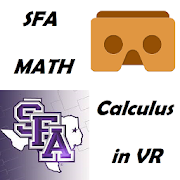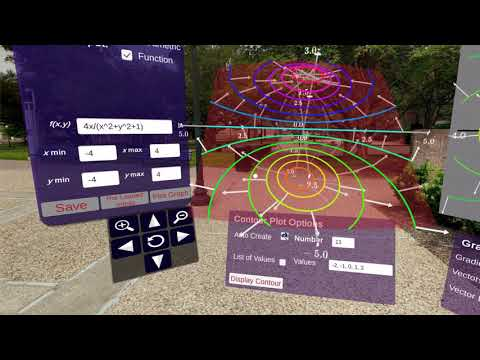Calculus in Virtual RealityEveryone
15Calculus in Virtual Reality (CalcVR)

Lessons about Calculus and Geometry in Virtual Reality!

We have added literal depth to calculus!
The CalcVR app uses a Google Cardboard headset to enable the user to visualize concepts in multi-variable calculus within a virtual reality setting. The user can specify their own objects for visualization as well go through lessons on the geometry and calculus of multi-variable functions and the corresponding surfaces. In addition to this, there are interactive demonstrations where the user can further explore the concepts covered in the lessons. Because these elements are rendered in a virtual the user can see the depth of these mathematical objects and the multiple facets at play in the study of these mathematics topics.
Users can use any Google Cardboard (v1.0, v2.0) or compliant viewer to work on lessons related to calculus and geometry in three dimensions. The headset should have a capacitive touch button or the user should use a Bluetooth controller
This app includes the following modules:

2D Coordinates and Graphs
- Rectangular 2D Coordinates
- Graphs and Sets
- Polar Coordinates (with two coordinate exploration demos)
- 2D Graph Demos (on conic sections, trigonometric functions, and lines in two dimensions)

3D Coordinates and Graphs
- Rectangular 3D Coordinates
- Lines and Planes (with two demos)
- Cylindrical Coordinates
- Spherical Coordinates
- 3D Coordinate Demos (with all three coordinate systems)
Curves and Surfaces
- Parametrizing Curves
- Parametrizing Surfaces
- Transformations of Surfaces
- Quadric Surfaces Demo
- Surface Plotting Demo (for parametric forms of surfaces)

Vector Valued Functions of 1 Variable
- Interactive Demo with user input (including dynamic vector and scalar calculations/visualizations)
- Intro to Vector Valued Functions
- Velocity and Speed
- Acceleration
- Unit Tangent Vector
- Unit Normal Vector
- Splitting of Acceleration
- Curvature
- The Binormal Vector

Vector Fields
- Vector Field Visualization Demo
- Plotting Vector Fields
- Divergence of a Vector Field
- Curl of a Vector Field

Multivariable Functions
-Plotting Multivariable Functions
-Contour Plots
-Limits and Continuity
-Partial Derivatives
-Directional Derivatives
-Tangent Planes and Linearity
-Extrema of Multivariable Functions
-Extrema on Compact Regions

Vector Calculus
-Line Integrals of Scalar Functions
-Line Integrals of Vector Fields
-Surface Integrals (Coming Soon)
Collapse

Review Policy
4.5
15 total
5
4
3
2
1

What's New

Improvements to several scenes in the vector valued functions of 1 variable lessons and demo.
Collapse

Updated
September 13, 2019
Size
134M
Installs
1,000+
Current Version
1.81
Requires Android
5.0 and up
Content Rating
Everyone
Permissions
Offered By
SFASU Math and Stat